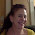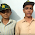## Monday, May 16, 2016

### Teaching probability with seven questions from the game of Monopoly.

Question One:  Players start the game of monopoly from Go and throw two dice.   The sum of the two dice rolls ranges from 2 to 12.   List all squares that the player may land on after one roll of the two dice.   What are the likelihoods of landing on each property after one roll of the two dice?

For a discussion of question one go to the following post:

Question Two:  Each turn of monopoly starts with a roll of two dice.   If the player rolls doubles (the same value on each dice) the person rolls again.   If the player rolls three doubles in a row the person goes directly to jail.   The player who rolls three doubles in a row does not get to buy a property or use a community chest or chance card.

The monopoly turn ends after the first roll of the dice if the dice roll is not doubles and after the third roll of the dice regardless of the dice roll outcomes.

The Chance Square exists seven squares away from GO.  What is the probability that a player starts as Go and lands on Chance on the first turn of the game?

For a discussion of question two go to the following post:

Question Three:  Sally owns electric company but does not own waterworks.   Mark lands on electric company.   The rent on electric company is 4 times the sum of two dice throws. What is the value of each possible amount that Mark will owe Sally and the probability of that outcome?  What is most likely amount that Mark will owe Sally?   What is the expected amount that Mark will owe Sally?

Sally buys waterworks and Mark lands on electric company again.   The rent is now 10 times the sum of the two dice rolls.   What is the most likely amount that Mark will owe Sally?   What is the expected amount?

In these two problems the most likely amount owed and the expected amount owed are equal.   Is this always the case?   Why or why not?

What is the most likely payoff from landing on electric company and the expected rent from landing on electric company if the rent is the square of the sum of the two dice rolls in dollars?

For a discussion of question three go to the following post:

Question Four:  What is the skewness of rent from electric company when rent is \$4.00 times the sum of the values from two dice rolls?

Would the skewness number change if rent is \$10 times the sum of the two dice rolls?

What is the skewness number if rent was equal to the square of the sum of the two dice rolls?

For a discussion of question four go to the following post.

Question Five: What is the probability that a person starts the game by throwing doubles on the first throw and then throws a second time and lands on Pennsylvania Railroad?   (Pennsylvania Railroad is the railroad that is 15 squares away from Go, the opening square.)

For a discussion of question five go here:

Question Six: A person starts on Kentucky Avenue and throws the dice once.   (We will consider ramifications of doubles in a future post but for now let’s assume the person does NOT go again if he throws doubles.)

The player’s opponent owns all three yellow properties --  Atlantic, Ventnor, and Marvin Gardens.    The rents at these three properties for two houses and for three houses are presented below.

 Rent at Atlantic, Ventor, and Marvin Gardens Two Houses Three Houses Atlantic 330 800 Ventor 330 800 Marvin Gardens 360 850

What is the likelihood that the person will not land on any of these three properties after one throw of the two dice?

What is the most likely outcome after one throw of the two dice?

What are the expected value, standard deviation, and skewness of rents given two houses and given three houses?

For a discussion of question six go to the following place.

Question:   A person rolls the two dice from Go.   After the roll of the two dice the player moves the dice back to Go and goes again.   What is the probability that at least one of the two rolls lands the person on chance, the square that is seven squares away from Go?

Hint:   The answer is NOT the sum of the probabilities from the first try and the second try.   Think of the probability of getting two heads on two coin tosses.   The answer is NOT the sum of the probabilities for the two coin tosses.

I hope that you and your students benefit from these problems.

1.2.